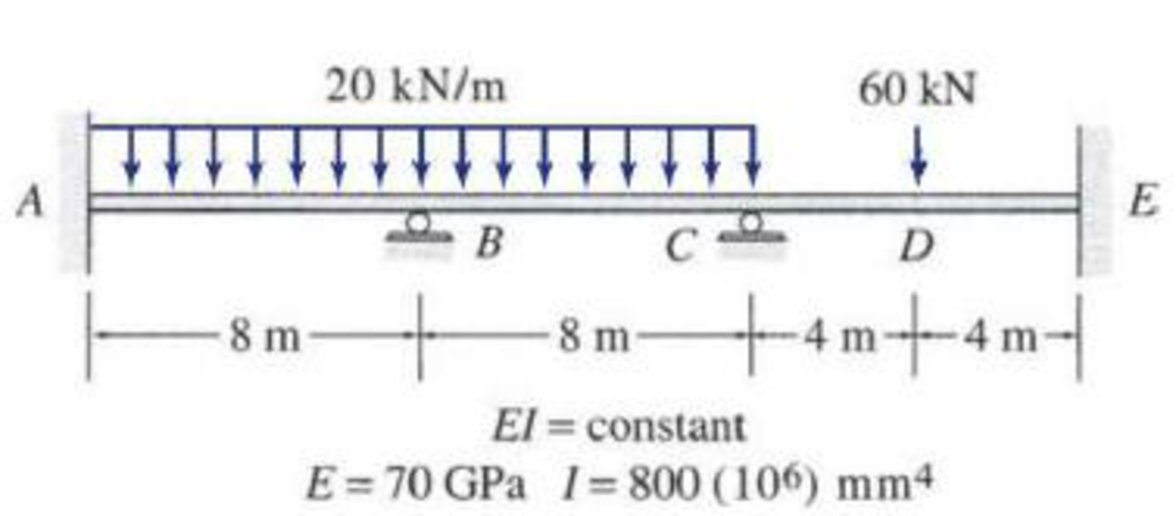# Determine the reactions and draw the shear and bending moment diagrams for the beams shown in Figs. P16.8–P16.14 by using the moment-distribution method. FIG. P16.9, P16.15

#### Solutions

Chapter
Section
Chapter 16, Problem 9P
Textbook Problem
164 views

## Determine the reactions and draw the shear and bending moment diagrams for the beams shown in Figs. P16.8–P16.14 by using the moment-distribution method.FIG. P16.9, P16.15

To determine

Find the reaction and plot the shear and bending moment diagram.

### Explanation of Solution

Fixed end moment:

Formula to calculate the relative stiffness for fixed support IL and for roller support (34)(IL).

Formula to calculate the fixed moment for point load with equal length are PL8.

Formula to calculate the fixed moment for point load with unequal length are Pab2L2 and Pa2bL2.

Formula to calculate the fixed moment for UDL is WL212.

Formula to calculate the fixed moment for deflection is 6EIΔL2

Calculation:

Consider flexural rigidity EI of the beam is constant.

Show the free body diagram of the entire beam as in Figure 1.

Refer Figure 1,

Calculate the relative stiffness KBA for member AB of the beam as below:

KBA=I8

Calculate the relative stiffness KBC for member BC of the beam as below:

KBC=I8

Calculate the relative stiffness KCB for member CB of the beam as below:

KCB=I8

Calculate the relative stiffness KCE for member CE of the beam as below:

KCE=I8

In the above beam joint B and C are free to rotate. Hence, calculate the distribution factor at joint B and C.

Calculate the distribution factor DFBA for member BA of the beam.

DFBA=KBAKBA+KBC

Substitute I8 for KBA and I8 for KBC.

DFBA=I8I8+I8=0.5

Calculate the distribution factor DFBC for member BC of the beam.

DFBC=KBCKBA+KBC

Substitute I8 for KBA and I8 for KBC.

DFBC=I8I8+I8=0.5

Check for sum of distribution factor as below:

DFBA+DFBC=1

Substitute 0.5 for DFBA and 0.5 for DFBC.

0.5+0.5=1

Hence, OK.

Calculate the distribution factor DFCB for member CB of the beam.

DFCB=KCBKCE+KCB

Substitute I8 for KCB and I8 for KEC.

DFCB=I8I8+I8=0.5

Calculate the distribution factor DFCE for member CE of the beam.

DFCE=KCEKCB+KCE

Substitute I8 for KCB and I8 for KCE.

DFCE=I8I8+I8=0.5

Check for sum of distribution factor as below:

DFCB+DFCE=1

Substitute 0.5 for DFCB and 0.5 for DFCE.

0.5+0.5=1

Hence, OK.

Calculate the fixed end moment for AB.

FEMAB=20×8212=106.7kNm

Calculate the fixed end moment for BA.

FEMBA=106.7kNm

Calculate the fixed end moment for BC.

FEMBC=106.7kNm

Calculate the fixed end moment for CB.

FEMCB=106.7kNm

Calculate the fixed end moment for CE.

FEMCE=60×88=60kNm

Calculate the fixed end moment for EC.

FEMEC=60kNm

Show the calculation of final moments using moment distribution method as in Table 1.

Show the section free body diagram of the member AB, BC and CE as in Figure 2.

Consider the member AB of the beam:

Calculate the vertical reaction at the left end of the joint B by taking moment about point A.

+MA=0By,L(8)20×(8)×(82)+103.6112.9=0By,L(8)=649.3By,L=649.38By,L=81.2kN

Calculate the horizontal reaction at point A by resolving the horizontal equilibrium.

+Fx=0Ax=0

Calculate the vertical reaction at point A by resolving the vertical equilibrium.

+Fy=0Ay(20×8)+By,L=0Ay160+81.2=0Ay=78.8kN

Consider the member BC of the beam:

Calculate the vertical reaction at the right end of the joint B by taking moment about point C

### Still sussing out bartleby?

Check out a sample textbook solution.

See a sample solution

#### The Solution to Your Study Problems

Bartleby provides explanations to thousands of textbook problems written by our experts, many with advanced degrees!

Get Started

Find more solutions based on key concepts
What are the major gases that make up the atmosphere?

Engineering Fundamentals: An Introduction to Engineering (MindTap Course List)

What are the two spindle types used for surface grinders?

Precision Machining Technology (MindTap Course List)

What is a table, and what role does it play in the relational model?

Database Systems: Design, Implementation, & Management

Describe the role of a driver. Explain how to find the latest drivers for a device.

Enhanced Discovering Computers 2017 (Shelly Cashman Series) (MindTap Course List)

What are optimizer hints, and how are they used?

Database Systems: Design, Implementation, & Management

If your motherboard supports ECC DDR3 memory, can you substitute non-ECC DDR3 memory?

A+ Guide to Hardware (Standalone Book) (MindTap Course List)

What is the purpose of grooving a joint before welding on thick plate?

Welding: Principles and Applications (MindTap Course List)